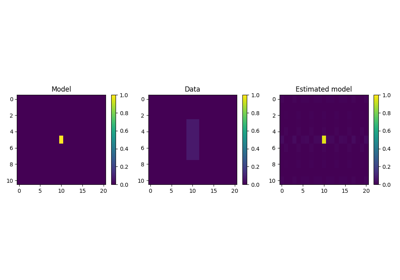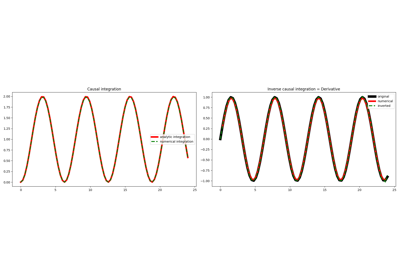# pylops.Smoothing2D¶

pylops.Smoothing2D(nsmooth, dims, axes=(-2, -1), dtype='float64')[source]

2D Smoothing.

Apply smoothing to model (and data) along two axes of a multi-dimensional array.

Parameters: nsmooth : Lenght of smoothing operator in 1st and 2nd dimensions (must be odd) dims : tuple Number of samples for each dimension axes : int, optional New in version 2.0.0. Axes along which model (and data) are smoothed. dtype : str, optional Type of elements in input array.

Notes

The 2D Smoothing operator is a special type of convolutional operator that convolves the input model (or data) with a constant 2d filter of size $$n_{\text{smooth}, 1} \times n_{\text{smooth}, 2}$$:

Its application to a two dimensional input signal is:

$y[i,j] = 1/(n_{\text{smooth}, 1}\cdot n_{\text{smooth}, 2}) \sum_{l=-(n_{\text{smooth},1}-1)/2}^{(n_{\text{smooth},1}-1)/2} \sum_{m=-(n_{\text{smooth},2}-1)/2}^{(n_{\text{smooth},2}-1)/2} x[l,m]$

Note that since the filter is symmetrical, the Smoothing2D operator is self-adjoint.

Attributes: shape : tuple Operator shape explicit : bool Operator contains a matrix that can be solved explicitly (True) or not (False)

## Examples using pylops.Smoothing2D¶2D SmoothingCausal Integration